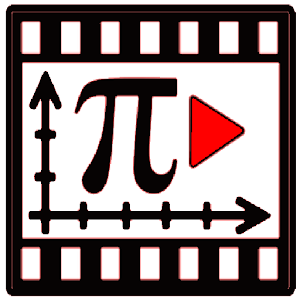## Fifth grade math - Graph points on a coordinate plane

Graphing points on a coordinate plane is important in understanding how two quantities depend on each other. To do this, we need to have knowledge of coordinate grid, quadrant numbers. Bar graph is another way of understanding distriubtion of numbers. You will find lot of 5 grade math problems for practice and 5th grade math test to gauge your understanding in the resouces available here. There are educational apps and videos explaining graphing points on a coordinate plane and math worksheets printable.

### The apps, sample questions, videos and worksheets listed below will help you learn Graph points on a Coordinate plane.

#### Educational Apps related to Graph points on a coordinate planeMath ELA Grade 5 – Common CoreGraphing Calculator | 5.in.1Math Course – calculus

#### Educational videos related to Graph points on a coordinate plane.

Report an Error